# Noise from numbers

## November 22, 2012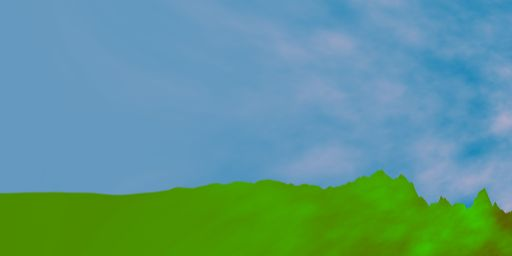Sometimes, a little noise is all you need to go from synthetic to organic.

The world is unpredictable, and that makes it interesting.

The same rules applies to shaders - unpredictable output makes for more interesting and natural-looking results. So how do you make a shader output unpredictable results?

An easy way is to feed it random numbers for every pixel in an image texture and use those in each calculation. But let's rule out that approach for now.

(It's anyway not always appropriate for complex shaders because a shader may end up doing many texture lookups from different parts of memory, and that can become a performance bottleneck).

Let's say you want the shader to generate unpredicatble output given smooth simple predicable input (and no textures).

To start with, there is no random number generator in GLSL. So you can't just call some built-in function like random() like you might on a CPU.

You need to provide your own function for this, one that takes any number as a parameter, mixes it up a bit, and returns another number in a known range (like between 0 and 1).

The output numbers for two close inputs should be very different so that when we show the output, we cannot see obvious patterns.

Unfortunately normal GLSL is also missing bit-level operations like shift and exclusive-or that would otherwise be very useful for making this kind of function.

Instead we need to make use of something that in other situations is annoying, but in this case will be useful - aliasing.

Aliasing is what happens when you sample a function at lower than double the frequency of the highest frequency component it contains. If you do that without filtering out the high frequencies first, they "reflect" and interfere with the rest of the signal.

When it happens with sound the result is often a ringing echo or sharpness. With images (say when you draw an image at half size using a "nearest neighbour" algorithm) it shows as sharp and pixellated edges or shimmering animation.

But today, for this problem, aliasing is going to be our friend by helping us to make some noise.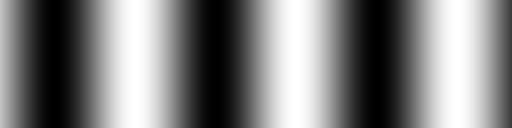```float r=.5+.5*sin(10.*c.x);
f=vec4(r)
```

To get aliasing, and potentially noise, we need to increase the frequency.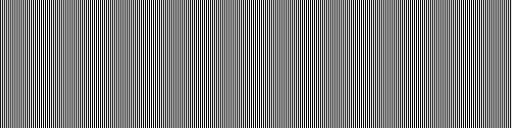```float r=.5+.5*sin(789.123*c.x);
f=vec4(r)
```

Hmm.. that didn't work. It is aliasing, but it's still giving us a regular pattern.

What we need is to combine it with another function - fract, which returns just the fractional part of a number, and as a side benefit will automatically give us a result in the range 0-1. This is essentially another repeating function which will show aliasing.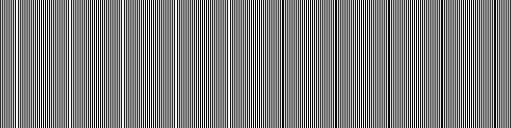```float r=fract(sin(789.123*c.x));
f=vec4(r)
```

Some signs of noise, but we're not there yet. We need to increase again the frequency of output of the fract, by increasing the amplitude of the sin.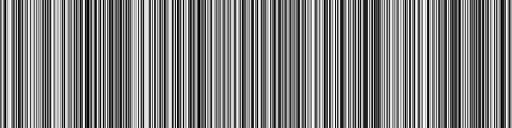```float r=fract(456.789*sin(789.123*c.x));
f=vec4(r)
```

Finally, some noise! Though it's only in 1-dimension. We need to try and turn this into 2D TV-static somehow.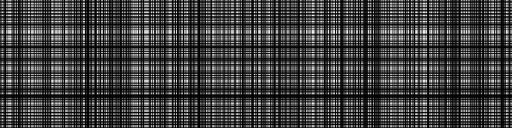```vec2 r=fract(456.789*sin(789.123*c.xy));
f=vec4(r.x*r.y)
```

Now we calculated the initial noise values based on both x and y coordinates. Then we combined the results by multiplying. But that wasn't very effective as the result has a clear pattern. Perhaps our fract is in the wrong place now?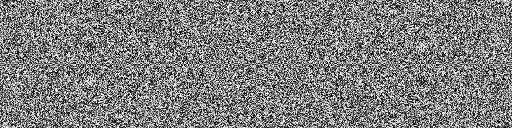```vec2 r=(456.789*sin(789.123*c.xy));
f=vec4(fract(r.x*r.y))
```

That's more like it. Now we have mostly the noise we were after, though there are still some hiddern patterns which brains do an amazing job at spotting. One cheap extra term should fix that.```vec2 r=(456.789*sin(789.123*c.xy));
f=vec4(fract(r.x*r.y*(1.+c.x)))
```

There, the patterns seem to have gone now. The final instruction tally per pixel with this approach is 2 sin, 1 fract, 6 multiplies and 1 add. That's not horrifyingly expensive, but sin can be a little painful.

Before this noise becomes useful for making images, we'll need to do some more work with it. But that's going to be the subject of another article.